SAS Technical Support occasionally receives requests from users who want to insert blank rows into their TABULATE procedure tables. PROC TABULATE syntax does not have any specific options that insert blank rows into the table results.

One way to accomplish this task is explained in SAS Sample 45972, "Add a blank row in PROC TABULATE output in ODS destinations." This sample shows you how to add a blank row between class variables in a PROC TABULATE table.  The sample code creates a new data set variable that you can use in a CLASS statement in the PROC TABULATE step.

In addition to the method that is shown this sample, there are two other methods that you can use to insert a blank row in PROC TABULATE table results. The following sections explain those methods:

### Method 1: Adding a blank row between row dimension variables

This section demonstrates how to add a blank row between row dimension variables.  This method expands on the approach that is used in Sample 45972.

In the following example, additional data-set variables are added to the data set in a DATA step. The BLANKROW variable is used as a class variable, and the variables DUMMY1 and DUMMY2 are used as analysis variables in the PROC TABULATE step. This sample code also uses the AGE and SEX variables from the SASHELP.CLASS data set as class variables in PROC TABULATE.

```/*** Method 1 ***/ data class; set sashelp.class; blankrow=' ';   dummy1=1; dummy2=.; run;   proc tabulate data=class missing; class age sex blankrow; var dummy1 dummy2; table age*dummy1=' ' blankrow=' '*dummy2=' '   sex*dummy1=' ' blankrow=' '*dummy2=' '   all*dummy1=' ',   sum*F=8. pctsum / misstext=' ' row=float;   keylabel sum='# of Students' pctsum='% of Total' all='Total';   title 'Add a Blank Row by Using New Data Set Variables'; run;```

This method produces the result that is shown below.  You can use ODS HTML, ODS PDF, ODS RTF, or ODS EXCEL statements to display the table.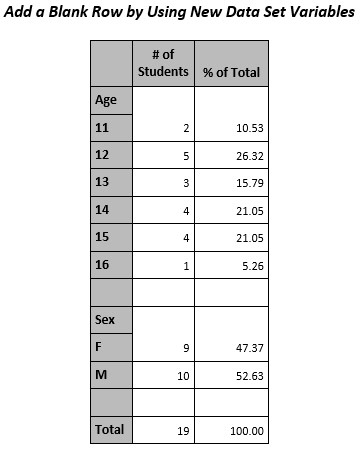### Method 2: Adding blank rows with user-defined formats and the PRELOADFMT option in the CLASS statement

The second method creates user-defined formats and uses the PRELOADFMT option in a CLASS statement in PROC TABULATE.  The VALUE statements that use the NOTSORTED option in the PROC FORMAT step establish the desired order of the results in the TABULATE results. Using the formats and specifying the ORDER=DATA option in the CLASS statement and the PRINTMISS option in the TABLE statement keeps the order requested in the PROC FORMAT VALUE statements and display the blank rows.

```/*** Method 2 ***/ proc format; value \$sexf (notsorted) 'F'='F' 'M'='M' ' '=' ';   value agef (notsorted) 11='11' 12='12' 13='13' 14='14' 15='15' 16='16' .=' ';   value \$sex2f (notsorted default=8) 'F'='F' 'M'='M' ' '='Missing' '_'=' ';   value age2f (notsorted) 11='11' 12='12' 13='13' 14='14' 15='15' 16='16' .=' .' 99=' ';   value mymiss 0=' ' other=[8.2];   run;   proc tabulate data=sashelp.class missing; class sex age / preloadfmt order=data;   table sex age all, N pctn*F=mymiss. / printmiss misstext=' ' style=[cellwidth=2in];   format sex \$sexf. age agef.; /* If there are no missing values for the class */ /* variables, use the formats \$SEXF and AGEF.*/ /* With missing values for the class variables, */ /* use the formats \$SEX2F and AGE2F. */   keylabel N='# of Students' PctN='% of Total' all='Total';   title 'Add a Blank Row by Using the PRELOADFMT Option'; run;```

This method produces the result that is shown below. You can use ODS HTML, ODS PDF, ODS RTF, or ODS EXCEL statements to display the table.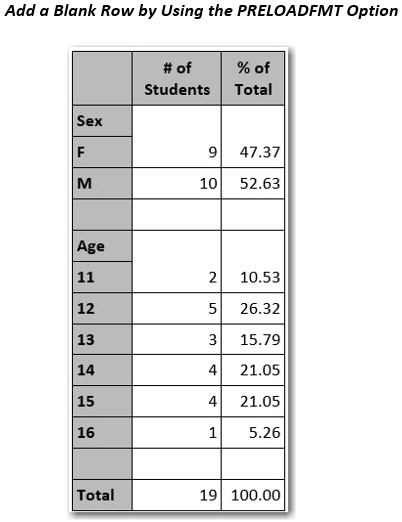If you have any questions about these methods, contact SAS Technical Support. From this link, you can search the Technical Support Knowledge Base, visit SAS Communities to ask for assistance, and contact SAS Technical Support directly.

Adding blank rows in TABULATE procedure results was published on SAS Users.During my 35 years of using SAS® software, I have found the CNTLIN and CNTLOUT options in the FORMAT procedure to be among the most useful features that I routinely suggest to other SAS users. The CNTLIN option enables you to create user-defined formats from a SAS data set (input control data set). The CNTLOUT option enables you to create a SAS data set (output control data set) containing format details from an entry in a SAS format catalog.

In this blog post, I provide a few examples demonstrating how to use the CNTLIN option. I also mention how to use the CNTLOUT option to store your format information in case you need to move to a new operating environment.

You can store all the format details from a SAS format catalog in a CNTLOUT data set and later restore them in a format catalog in your new operating environment using the CNTLIN option. For details, see SAS Usage Note 22194: “How to use the CNTLOUT= and CNTLIN= options in PROC FORMAT to move formats from one platform to another.”

A data set for the CNTLIN option contains variables that give specific information about ranges and values. At a minimum, the data set must contain the following variables:

FMTNAME specifies a character variable whose value is the format or informat name.
START specifies a variable that gives the range's starting value.
LABEL specifies a variable whose value is associated with a format or an informat.

For details about input and output control data sets, see the “FORMAT Procedure” section of Base SAS® 9.4 Procedures Guide, Seventh Edition.

### Create a Numeric Format

The following simple example using the CNTLIN option creates a numeric format named respf:

``` data test; input response desc \$20.; datalines; 1 Strongly Disagree 2 Disagree 3 Neutral 4 Agree 5 Stongly Agree ; run;   data crfmt; set test; start=response; label=desc; fmtname='respf'; run;   proc format library=work cntlin=crfmt fmtlib; select respf; run;```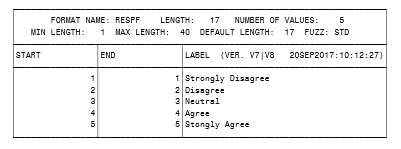### Reveal Data Set Variables

To see the other variables that are included in data sets created by the CNTLIN and CNTLOUT options, use CNTLOUT to create a data set for the respf format created above:

``` proc format library=work cntlout=outfmt; select respf; run; proc print data=outfmt; run;```

### Add Additional Ranges

To add another range to the respf format, you can use DATA step processing with the data set created by the CNTLOUT option. Then, re-create the format using the CNTLIN option:

```data infmt; set outfmt end=last; output; if last then do; HLO='O'; /* indicates a special other range */ label='NA'; output; end; run;   proc format library=work cntlin=infmt fmtlib; select respf; run;```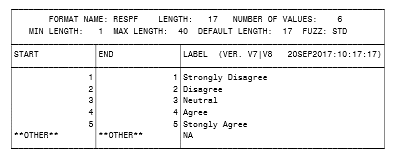### Convert a State Name to Its Postal Abbreviation

One use for the CNTLIN option is to create a format that converts a state name to its 2-letter postal abbreviation. For example, this option can convert 'North Carolina' to 'NC'.  Because SAS does not have a function or format to convert state names to postal abbreviations, this is an excellent use of the CNTLIN option.

We can use data from the SASHELP.ZIPCODE data set to create a user-defined format using the CNTLIN option, as shown below:

```proc sql noprint; create table crfmt as select distinct statename as start, statecode as label, '\$mystate' as fmtname from sashelp.zipcode; quit;   proc format library=work cntlin=crfmt fmtlib; select \$mystate; run;```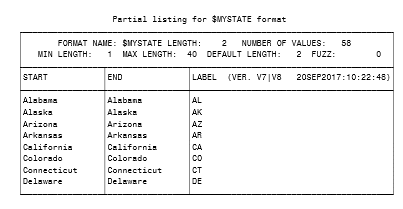### Identify State Capitals

In a similar manner, we can use the MAPS.USCITY data set to create a user-defined format that identifies state capitals from the 2-letter state abbreviation. See the sample code and partial results below:

```proc sql noprint; create table crfmt as select distinct statecode as start, city as label, '\$mycity' as fmtname from maps.uscity where capital='Y'; quit;   proc format library=work cntlin=crfmt fmtlib; select \$mycity; run;```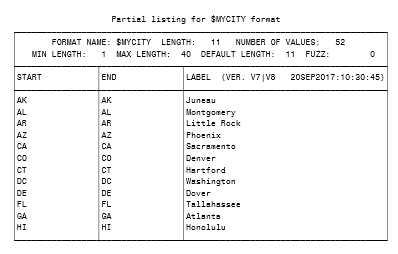### Use External Data Sources

You can gather information from external data sources and read that information into a data set created by the CNTLIN option to create user-defined formats.

The following example uses ICD10 medical diagnosis codes. I downloaded a list of ICD10 codes and their descriptions into a Microsoft Excel file from the Center for Medicare & Medicaid Services website. Then, I created a user-defined format from the first 25 records:
Note: You can also download the codes as a text file.

```/* This code reads in the Excel file. */ proc import out==myicd10 datafile= "C:\Section111ValidICD10-2017.xlsx" dbms=excelcs replace; range="'Valid ICD10 2017 &amp; NF Exclude\$'"; scantext=yes; usedate=yes; scantime=yes; run;     data crfmt; set myicd10 (obs=25); fmtname='\$myicd'; start=code; label=short_description; run;   title1 'ICD10 Format'; title3 'FMTLIB results only display the first 40 characters of the label'; proc format library=work cntlin=crfmt fmtlib; select \$myicd; run;```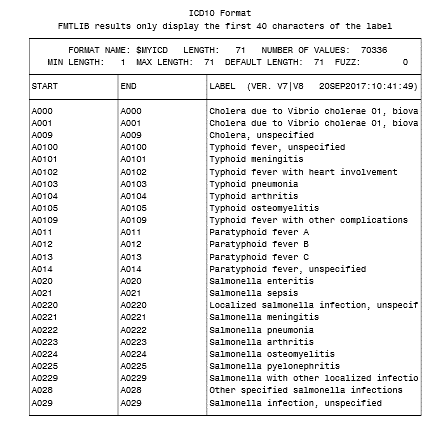A more complicated example that uses other data set variables created by the CNTLIN option is included in the linked sample program in Sample 47312: “Create a user-defined format containing decile ranges from PROC UNIVARIATE results.”

If you can think of a scenario in which the CNTLIN format would be helpful, give it a try. If you have questions, you can ask via  SAS Communities or contact us in SAS Technical Support.

Controlling your formats was published on SAS Users.SAS users often ask me about the best way to group or bin their data in preparation for additional analysis. Depending on the need, there are several ways to achieve this using SAS procedures or other SAS processing. Creating user-defined formats with PROC FORMAT or using Data step processing are two of the methods that are commonly used.

Often, users need to generate quantile rankings based on the values to create quantile groups like quartiles, quintiles, or deciles. It can be a memory-intensive procedure, but the syntax is pretty simple. For example, you can create quintile groups by specifying GROUPS=5 in the PROC RANK statement. The variable named in the RANKS statement will contain values ranging from 0 to 4 for the groups in the output data set. The following code creates a test data set and shows a simple PROC RANK step. A PROC REPORT step was used to display the results.

```data test; /*  Creating a sample data set  */
do i=1 to 99;
xyz=round((ranuni(i)*1234),.1);
output;
end;
drop i;
run;

proc rank data=test out=out1 groups=5;
var xyz;
ranks rank1;
run;
```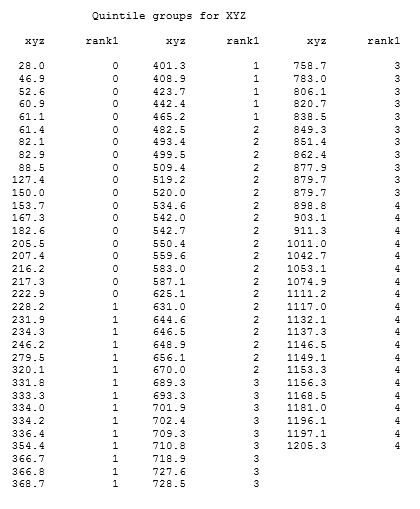The formula PROC RANK uses to create the groups is:

```    group=floor(rank* k/(n+1));

where:
FLOOR is the FLOOR function
rank is the value's order rank. That is the observation number in sort order,
but, for duplicate(tied) values, it's the average observation number.

k is the value of GROUPS=
n is the number of observations having nonmissing values of the ranking variable

```

We can duplicate the results from PROC RANK via a couple of PROC steps and Data step processing using this formula. Using the test data set from above, the following steps create the variables to be used in the formula and applies the formula in a final Data step. The results are the same as those generated by PROC RANK.
```proc sort data=test;
by xyz;
run;

data test2;
set test;
obsnum=_N_;
run;

proc means data=test2 NWAY noprint;
class xyz;
var obsnum;
output out=out2 (drop=_type_ _freq_) mean=order_rank;
run;

proc print data=out2;
run;

data final;
merge test2 out2;
by xyz;
rank2=floor(order_rank *(5/100));
drop obsnum;
/*  floor(rank* k/(n+1));  */
/*  rank=order_rank,  k=5 groups,  n=99 observations */
run;

proc print data=final;
var xyz rank2 order_rank;
run;
```

The GROUPS= option generates quantile ranks. If the data is skewed, that is, if the values of the variable being ranked are not evenly distributed, PROC RANK may not generate the number of groups requested nor groups of equal size. In such cases, there is no option within PROC RANK to ensure that you get the requested number of groups nor groups of equal size.

The following program shows a case when only five groups are generated when GROUPS=10 was requested. You’ll see that the majority of the values are 0. You can look at the decile ranks in the PROC UNIVARIATE output data set to see that the first six deciles generate the same value, 0. Using the formula from above, PROC RANK assigns the 0 values to one group and the other values to 4 other groups.

```data test;  /*  creating sample data set with mostly 0 values */
do i=1 to 40;
x=int(ranuni(i)*44);
output;
end;
do i=41 to 105;
x=0;
output;
end;
run;

proc rank data=test out=test2 groups=10;
var x;
ranks rx;
run;

proc freq data=test2;
tables rx / nopercent nocum;
run;

proc univariate data=test noprint;
var x;
output out=outdec pctlpre=P pctlpts=10 to 100 by 10;
run;

proc print data=outdec;
title 'Deciles for variable x ';
run;
```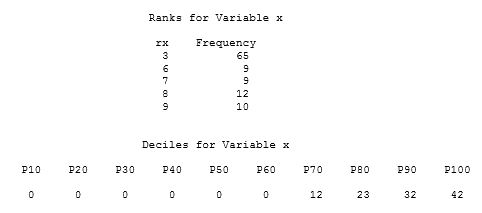PROC RANK creates the quantile groups (ranks) in the data set, but users often want to know the range of values in each quantile. There are no options in PROC RANK to determine those ranges. Using other program logic, we can determine those ranges and create a user-defined format containing the ranges.

You can use PROC UNIVARIATE or PROC MEANS to create an output data set containing quantile values for the variable to be ranked. After transposing the output data set from UNIVARIATE or MEANS, DATA step processing is used to create a data set that can be used in the CNTLIN= option of PROC FORMAT. The user-defined format created using PROC FORMAT can then be used in a DATA step or procedure step. An existing Sample showing this method is available at the following link.

Sample 47312: Create a user-defined format containing decile ranges from PROC UNIVARIATE results.

Creating Quantile Groups was published on SAS Users.